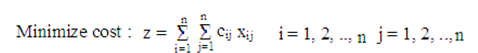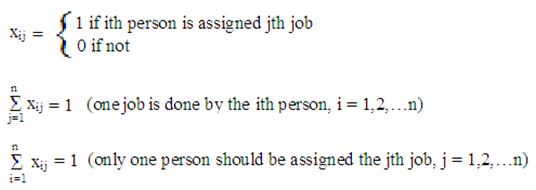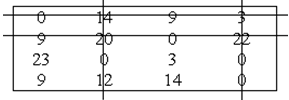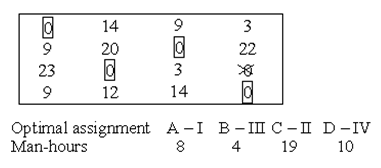#### Introduction to Assignment Problem & Algorithm for Assignment Problem

Introduction to Assignment Problem

In assignment problems, the aim is to allot a number of jobs to the equivalent number of persons at a least cost of maximum profit.

Assume there are 'n' jobs to be done and 'n' persons are available for completing these jobs. Suppose each person can do each job in a time with a varying or fluctuating degree of efficiency. Assume cij be the cost of ith person allotted to jth job. Then the problem is to discover an assignment in a way that the total cost for doing all jobs is least. These problems are called as assignment problems.

These problems may comprise of classes to the rooms, assigning men to offices or problems to the research team etc.

Mathematical formulation or equation

Cost matrix: cij=   c11    c12    c13   ...   c1n

c21    c22     c23   ...   c2n

.

.

.

cn1     cn2    cn3  ...   cnnSubject to restrictions of the formWhere xij symbolizes that jth job is to be allotted to the ith person.

This exclusive structure of assignment problem permits a more easy method of solution as compared to simplex method.

Algorithm for Assignment Problem (Hungarian Method)

Step 1

Subtract the minimum of every row of the efficiency matrix, from all the elements of the subsequent rows (Row reduced matrix).

Step 2

Then change the resultant matrix by subtracting the minimum element of each column with all the elements of the respective columns. Hence first modified matrix is achieved.

Step 3

Make the minimum number of horizontal and vertical lines to cover up all the zeroes in the resultant matrix. Assume the minimum number of lines be N. Then there may be two possibilities

• If N = n, the number of rows (columns) of the given matrix then a best assignment can be prepared. Thus make the zero assignment to obtain the necessary solution.
• If N < n then go for step 4

Step 4

Find out the least element in the matrix, not covered by N lines. Subtract this minimum element with all uncovered elements and add the identical element at the intersection of vertical and horizontal lines. Therefore the second modified matrix is achieved.

Step 5

Repeat step 3 and step 4 again and again until minimum number of lines turns out to be equal to number of rows (columns) of the given matrix i.e. N = n.

Step 6

To prepare zero assignment - test the rows successively until a row-wise precisely single zero is found; mark this zero with '1''to prepare the assignment. Then, mark an 'X' over all zeroes if staying in the column of the marked zero, showing that they cannot be taken for subsequent assignment. Repeat in this manner until all the rows have been checked. Continue the similar process for the columns as well.

Step 7

Repeat the step 6 consecutively until one of the following situations occur

• If no unmarked zero is left, then end the process
• If there stays more than one of the unmarked zeroes in any of the column or row, then mark '1''one of the unmarked zeroes randomly and put a cross in the cells of remaining zeroes in its row and column. Continue this process until no unmarked zero is left in the matrix.

Step 8

Exactly one marked zero in each column and each row of the matrix is achieved. The assignment matching to these marked zeroes will provide the optimal assignment.

Example 1

A department head has four subordinates and four jobs need to be done. Subordinates vary in efficiency and tasks differ in their intrinsic complexity. Time each man would require to complete each task is given in the effectiveness matrix. How the tasks should be assigned to each person in order to minimize the total man-hours?

 Tasks Subordinates I II III IV A 8 26 17 11 B 13 28 4 26 C 38 19 18 15 D 19 26 24 10

Row Reduced Matrix

 0 18 9 3 9 24 0 22 23 4 3 0 9 16 14 0

I Modified MatrixN = 4, n = 4

As N = n, we proceed to zero assignment

Zero assignmentTotal man-hours = 8 + 4 + 19 + 10 = 41 hours